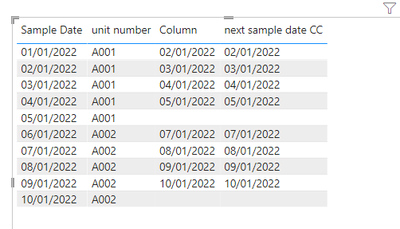cancel
Showing results for
Did you mean:

Fabric is Generally Available. Browse Fabric Presentations. Work towards your Fabric certification with the Cloud Skills Challenge.Helper III

## How to get value of the next row for a certain category?

I have been able to create a calculated column using the EARLIER function, however I am unsure on how to find the next row (or more than 1 row earlier for that matter).

The DAX below is what I have used to find the previous Sample Date for the same unit number. Can someone please tell me how I would find the next sample date and if there is a better way of writing my current formula? I feel like using MAX or SUM here is a little dodgy as I am not actually finding either, I just want to return a single value.

``````=
CALCULATE
(
MAX
(
[Sample Date]
),
FILTER
(
'Sample Data',
'Sample Data'[Sample Date] < EARLIER
(
'Sample Data'[Sample Date]
)
),
'Sample Data'[Unit Number] = EARLIER
(
'Sample Data'[Unit Number]
)
)``````

1 ACCEPTED SOLUTIONSuper User

Or if you want  more generic solution, , where you can specify the poisition you want by changing N_  (4th last in the example):

``````A =
VAR N_ = 4 // 1 is the immediately previous, 2 is the one before and so forth
VAR auxT_ =
TOPN (
N_,
CALCULATETABLE (
DISTINCT ( 'Sample Data'[Sample Date] ),
'Sample Data'[Sample Date] < EARLIER ( 'Sample Data'[Sample Date] ),
ALLEXCEPT ( 'Sample Data', 'Sample Data'[Unit Number] )
),
'Sample Data'[Sample Date], DESC
)
RETURN
MINX ( auxT, 'Sample Data'[Sample Date] )
``````Please accept the solution when done and consider giving a thumbs up if posts are helpful.  Contact me privately for support with any larger-scale BI needs, tutoring, etc.

5 REPLIES 5Community Support

Hi @justlogmein ,

``````Column =
CALCULATE (
MIN ( 'Sample Date'[Sample Date] ),
FILTER (
'Sample Date',
'Sample Date'[Sample Date] > EARLIER ( 'Sample Date'[Sample Date] )
&& 'Sample Date'[unit number] = EARLIER ( 'Sample Date'[unit number] )
)
)
``````Or try to use @Jihwan_Kim 's way.

If I have misunderstood your meaning, please provide your pbix file without privacy information and desired output.

Best Regards

Community Support Team _ Polly

If this post helps, then please consider Accept it as the solution to help the other members find it more quickly.Super User

Hi,

Please try the below for creating a new column.

``````next sample date CC =
MINX (
FILTER (
'Sample Data',
'Sample Data'[Sample Date] > EARLIER ( 'Sample Data'[Sample Date] )
&& 'Sample Data'[Unit Number] = EARLIER ( 'Sample Data'[Unit Number] )
),
'Sample Data'[Sample Date]
)
``````

If this post helps, then please consider accepting it as the solution to help other members find it faster, and give a big thumbs up.Super User

``````Top 1 Unit Number =
VAR CategoryTable =
CALCULATETABLE (
'Sample Data',
ALLEXCEPT ( 'Sample Data', 'Sample Data'[Category] )
)
VAR Top1Value =
MAXX ( CategoryTable, 'Sample Data'[Sample Date] )
VAR Top1UnitNumber =
MAXX (
FILTER ( Top2Table, 'Sample Data'[Sample Date] = Top1Value ),
'Sample Data'[Unit Number]
)
RETURN
Top1UnitNumber``````
``````2nd Top Unit Number =
VAR CategoryTable =
CALCULATETABLE (
'Sample Data',
ALLEXCEPT ( 'Sample Data', 'Sample Data'[Category] )
)
VAR Top2Table =
TOPN ( 2, CategoryTable, 'Sample Data'[Sample Date] )
VAR Top2Value =
MINX ( Top2Table, 'Sample Data'[Sample Date] )
VAR Top2UnitNumber =
MAXX (
FILTER ( Top2Table, 'Sample Data'[Sample Date] = Top2Value ),
'Sample Data'[Unit Number]
)
RETURN
Top2UnitNumber``````Super User

Or if you want  more generic solution, , where you can specify the poisition you want by changing N_  (4th last in the example):

``````A =
VAR N_ = 4 // 1 is the immediately previous, 2 is the one before and so forth
VAR auxT_ =
TOPN (
N_,
CALCULATETABLE (
DISTINCT ( 'Sample Data'[Sample Date] ),
'Sample Data'[Sample Date] < EARLIER ( 'Sample Data'[Sample Date] ),
ALLEXCEPT ( 'Sample Data', 'Sample Data'[Unit Number] )
),
'Sample Data'[Sample Date], DESC
)
RETURN
MINX ( auxT, 'Sample Data'[Sample Date] )
``````Please accept the solution when done and consider giving a thumbs up if posts are helpful.  Contact me privately for support with any larger-scale BI needs, tutoring, etc.Super User

``````NewCol =
VAR previousDate_ =
CALCULATE (
MAX ( [Sample Date] ),
'Sample Data'[Sample Date] < EARLIER ( 'Sample Data'[Sample Date] ),
ALLEXCEPT ( 'Sample Data', 'Sample Data'[Unit Number] )
)
RETURN
CALCULATE (
MAX ( [Sample Date] ),
'Sample Data'[Sample Date] < previousDate_,
ALLEXCEPT ( 'Sample Data', 'Sample Data'[Unit Number] )
)
``````Please accept the solution when done and consider giving a thumbs up if posts are helpful.  Contact me privately for support with any larger-scale BI needs, tutoring, etc.Announcements#### Power BI Monthly Update - November 2023

Check out the November 2023 Power BI update to learn about new features.#### Fabric Community News unified experience

Read the latest Fabric Community announcements, including updates on Power BI, Synapse, Data Factory and Data Activator.#### The largest Power BI and Fabric virtual conference

130+ sessions, 130+ speakers, Product managers, MVPs, and experts. All about Power BI and Fabric. Attend online or watch the recordings.Top Solution Authors
Top Kudoed Authors
Users online (2,395)